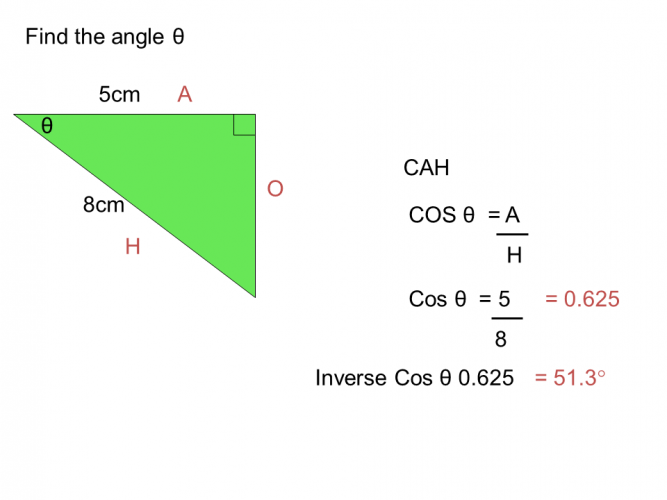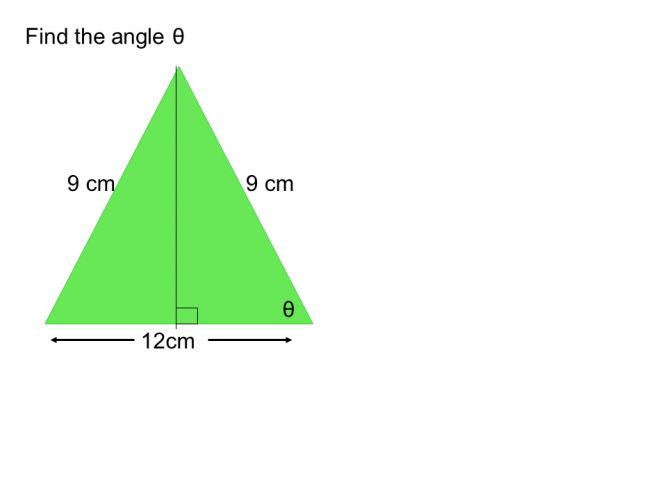# Find a Missing Angle Using the Cos Ratio

In this worksheet, students will use the Cos ratio to find the missing angle of a right angled triangle.Key stage:  KS 4

GCSE Subjects:   Maths

GCSE Boards:   AQA, Eduqas, OCR, Pearson Edexcel

Curriculum topic:   Geometry and Measures, Mensuration

Curriculum subtopic:   Mensuration and Calculation, Triangle Mensuration

Difficulty level:### QUESTION 1 of 10

Its all Greek to meDid you know that the word trigonometry came from the ancient Greeks.  'Trigonon' was their word for triangle and 'metron' their word for measure.

Don't you think these ancient Greeks were a bit geeky?I like being a maths geek.  People think I am really clever, but it is just a case of using formulas that the ancient Greeks gave us years ago... makes us look good.

(SOH, CAH, TOA) yes that again.

We are going to look at Cos here.

We can use Cos to help us find the missing angles in a  right angled triangle.

A formula triangle is helpful.  The stuff in the triangle is going to help us.

C is for Cos (which will be given as an angle)

H is the hypotenuse

The line in the middle means divide.To use this triangle we cover up what it is we want to find and we are left with a formula to follow.

Who would have thought formulas could be so helpful.We want to find the angle, cover up the C.  The formula we are left with is adjacent ÷ hypotenuseLet's give it a go.

IMPORTANT NOTE:  Make sure your calculator is set to degrees.  You should see a D in your window.

Example 11. Label the triangle

2. Find the two sides you want.

You want to find the angle, so we want A, and the only other side you have to help is the H.

3. Look at your formula triangle, cover up C

4.  We are left with Adjacent ÷ Hypotenuse

5. In your calculator type in  3 ÷ 7 = 0.42 (This is clearly wrong)

This is the geeky bit

6. Type into your calculator Shift Cos to access Cos-1  0.42 which will give us the angle.

Example 21. Label the triangle

2. Look at your formula triangle, cover up C

4.  We are left with Adjacent ÷ Hypotenuse

5. In your calculator type in  5 ÷ 8 = 0.625

6. Do the geeky bit Shift Cos to access Cos-1  0.625 which will give us the angle.

Clever things these calculators, not geeky after all..but still makes us look good.Answer has been rounded to 2 decimal places

35.21°

46.72°

48.19°

39.67°adjacent x hypotenuse adjacent ÷ hypotenuse 63.36° 68.68° 65.55° The formula I need is The angle isCalculate the size of the angle.

 adjacent x hypotenuse adjacent ÷ hypotenuse 63.36° 68.68° 65.55° The formula I need is The angle is22.25°

21.78 °

27.95°

20.69°adjacent x hypotenuse adjacent ÷ hypotenuse 60° 65° 55° The formula I need is the angle isadjacent x hypotenuse adjacent ÷ hypotenuse 60° 65° 55° The formula I need is the angle isAnswer has been rounded to 2 decimal places (2 d.p)

48.19°

46.23°

45.67°

47.20°

• Question 1Answer has been rounded to 2 decimal places

48.19°
EDDIE SAYS
The tricky part of the question is labelling your triangle correctly. Why do you have to label the triangle? Just be cos.... You should have discovered that you needed the adjacent divided by the hypotenuse. 8 ÷ 12 = 0.6666 cos-1 0.666 = 48.19 (to 2 d.p)
• Question 233.6
EDDIE SAYS
How are you going with the labelling of the triangle? It can get tricky I know. Adjacent ÷ hypotenuse 5 ÷ 6 = 0.833 cos-1 0.833 = 33.59 (33.6° to 1 d.p)
• Question 3adjacent x hypotenuse adjacent ÷ hypotenuse 63.36° 68.68° 65.55° The formula I need is The angle is
EDDIE SAYS
Why didn't the sin and tan get on? Just cos... Have you noticed that the formula will always be the same when finding an angle, unlike when trying to find a side. 8 ÷ 22 = 0.3636 Cos-1 0.3636 = 68.68° to 2 decimal places
• Question 4Calculate the size of the angle.

EDDIE SAYS
It is like anything. Once you know the formula, or know how to find it, things become easy as it is just following a set of instructions. 7 ÷ 8 = 0.875 Cos-1 0.875 = 28.96°
• Question 520.69°
EDDIE SAYS
Are you getting used to this now? It is just a case of keep practicing finding and using the formula. 14.5 ÷ 15.5 = 0.935.. Cos-1 0.935 = 20.69° (2 d.p).
• Question 636.87
EDDIE SAYS
12 ÷ 15 = 0.8 Cos-1 0.8 = 36.87° (to 2 d.p) Top tip. Scientific calculators vary slightly. Make sure you always use the same one so you can get used to their ways.
• Question 7adjacent x hypotenuse adjacent ÷ hypotenuse 60° 65° 55° The formula I need is the angle is
EDDIE SAYS
To be honest once you can label your triangle correctly you can leave the rest to your scientific calculator. What a great friend it is at a time like this. 9 ÷ 18 = 0.5 Cos -1 0.5 = 60°
• Question 8EDDIE SAYS
Calculators are great, they are us look so clever. The only thing you have to be careful of, is rounding to the correct number of decimal places. 2.5 ÷ 6.5 = 0.3846... Cos-1 0.3846... = 67.38° (to 2 d.p)
• Question 963.61
EDDIE SAYS
Nearly there. I hope you haven't found this to tri-ing!! 4 ÷ 9 = 0.444 Cos-1 0.444 = 63.61° (to 2 d.p)
• Question 10Answer has been rounded to 2 decimal places (2 d.p)

48.19°
EDDIE SAYS
And finally, just cos...... You must have been expecting this, a non right angled triangle. What do you do..turn it into a right angled triangle of course. Don't forget to halve the base to get the correct measurement for the adjacent side. 6 ÷ 9 = 0.66666 Cos-1 48.19° (to 2 d.p)
---- OR ----

Sign up for a £1 trial so you can track and measure your child's progress on this activity.

### What is EdPlace?

We're your National Curriculum aligned online education content provider helping each child succeed in English, maths and science from year 1 to GCSE. With an EdPlace account you’ll be able to track and measure progress, helping each child achieve their best. We build confidence and attainment by personalising each child’s learning at a level that suits them.

Get started